Next: Contents Up: ALIAS-C++ Previous: Examples of application of

• La page de J-P. Merlet
• La page Présentation de HEPHAISTOS
• La page "Présentation" de l'INRIA

Subsections

# Compilation problems

Compilation problems may occur:
1. if you are using the Gnu C++ compiler with a version higher than 2.95 (the code of BIAS/Profil has not yet been modified to accommodate the new standard)
2. if you try to compile the interval evaluation of very complex expressions. Typically you may get a message indicating that the compiler has exhausted its virtual memory. C++ seems to be indeed quite sensitive to the size of the expressions.
To solve problem 2 a first possibility is to turn off the optimization flag that is used for the compilation and instead use -g as the compilation flag.

If the compilation cannot still be completed you are confronted to a difficult problem. Most probably the compilation problems are due to the size of the expressions that you are manipulating for your problem. A first solution is to try to simplify the generated expression using auxiliary variables for terms that appear at least twice in the code. ALIAS-Maple proposes a mechanism that may allow to perform part of this task automatically. But many time it will be still necessary to edit by hand the C++ program. If you are using ALIAS-Maple the procedure involving the expressions, their jacobian and Hessian are named usually F, J and H respectively and are located usually in the files _F_.c, _J_.c, _H_.c.

Another possibility is to compile separately the files that involves the interval evaluation of your expressions that you may have included directly in your main program.

This is the case for the C++ code produced by ALIAS-Maple which include the expressions files in the main program. Let us consider the example of the general solving procedure. The main program is named _GS_.C and is compiled with the makefile _makefile. This main program includes the expressions evaluation file _F_.c and eventually the jacobian and hessian evaluation files (_J_.c , _H_.c). To compile separately the expressions evaluation file first rename this program _F_.C and add the following header:

```
#include <fstream>
#include "Functions.h"
#include "Vector.h"
#include "IntervalVector.h"
#include "IntervalMatrix.h"
#include "IntervalMatrix.h"
#include "IntegerVector.h"
#include "IntegerMatrix.h"
```
and remove the #include "_F_.c" in the main program _GS_.C.

Then the makefile should be modified so that _F_.o will appear in the dependency. Hence the line:

```
_GS_:_GS_.C \$(LIB_SOLVE)
```
will be changed to:
```
_GS_:_GS_.C _F_.o \$(LIB_SOLVE)
```
Then indicate that _F_.o should be linked by adding this file name before the library flag LIB_SOLVE. Hence:
```
\$(CC) -I\$(INCL) -I\$(INCLI) _GS_.C -o _GS_ \
\$(LIB_SOLVE) -L\$(LIB) \$(LD) -lm
```
will be changed to:
```
\$(CC) -I\$(INCL) -I\$(INCLI) _GS_.C -o _GS_ \
_F_.o \$(LIB_SOLVE) -L\$(LIB) \$(LD) -lm
```

If the previous method does not work, then there is an alternative that will always work but at the expense of an increased computation time: instead of compiling your expression you may rather use the ALIAS-C++ parser to interpret all the expressions (see chapter 12). Basically you will have to write the analytical form of each expression you will have to evaluate in a text file and execute specific procedures of the parser library that will perform the interval evaluation of these expressions by reading this text file. Note that ALIAS-Maple is able to produce code that performs this operation (see the MakeF, MakeJ, MakeH procedures.

# Execution problems

## Interval valuation problems

You have now successfully completed the compilation of your program and you run it. But it just crashes with an error message such as:

```
BIAS Error: ArcSin argument out of range
BIAS Error: Power: Negative or zero exponent with zero base
BIAS Error: Division by Zero
```
You have to remember that not all expression may have an interval evaluation according to the ranges of the unknowns (see section 2.1.1.3 for a complete list of the expression that may lead to an evaluation problem.

To solve this problem first consider the expressions you have, determine which of them may lead to an evaluation problem and examine if you may modify the expression to avoid the interval valuation problem (for example for an equation whose terms have a common denominator just consider the numerator of the expression as the interval evaluation of the denominator may include 0, a typical case for which an interval valuation cannot be calculated).

If the problem persist you will have to identify which terms may lead to an interval valuation problem for each expression (we will call them the faulty terms). Then before performing the interval evaluation of the expression you will have to compute the interval evaluation of the faulty terms. If this evaluation leads to interval that will cause an evaluation problem, then instead of performing the interval evaluation of the expression you will affect an interval to the expression that depends on the context.

For example assume that you have to solve the equations system

```
y/(x-1)+cos(y)=0
arcsin(y)+x*y-1=0
```
with x, y in the range [-10,10]. The faulty term for the first equation is x-1 whose interval evaluation cannot include 0 (as we divide by x-1). The faulty term for the second equation is y which must lie in the range [-1,1] (as we have arcsin(y)). Now you want to write the procedure that will be used to compute the interval evaluation of these equations that is to be used by the general solving procedure. Initially you will have written this procedure as:
```
INTERVAL_VECTOR F(int l1,int l2, INTERVAL_VECTOR &X)
{
INTERVAL_VECTOR V(2);
if(l1==1)
V(1)=X(2)/(X(1)-1)+Cos(X(2));
if(l2==2)
V(2)=ArcSin(X(2))+X(1)*X(2);
return V;
}
```
In that case the procedure will crash immediately as for the initial search domain the first equation cannot be evaluated. Now if the interval evaluation of the faulty term of the first equation includes 0 we must not evaluate the equation. But still we wish to eliminate a box for which the interval evaluation of x-1 includes 0 only if the maximal absolute value of the interval evaluation is very close to 0. We may deal with this problem with the following code:
```
if(l1==1)
{
U=X(1)-1;
if(Sup(IAbs(U))<1.e-4)V(1)=1;
else
{
if(Inf(U)<=0 && Sup(U)>=0)V(1)=INTERVAL(-1.e8,1.e8);
else V(1)=X(2)/U+Cos(X(2));
}
}
```
If the interval evaluation of x-1 has a maximal absolute value lower than 1e-4 the interval evaluation of the first equation is 1 and the solving algorithm will discard the corresponding box. On the other hand we set the interval evaluation of the equation to an arbitrary interval that includes 0 so that the solving algorithm will not discard the box.

For the second equation the management of the evaluation problem is somewhat different. If the interval of y has no intersection with the interval [-1,1] then we may discard the box. Otherwise we may change the the interval for y to this intersection and proceed with the evaluation of the equation. To compute this intersection we use the Intersection procedure of BIAS/Profil that returns 0 if two intervals have an empty intersection. The code is

```
if(l2==1)
{
if(Intersection(U,X(2),INTERVAL(-1,1))==0)V(2)=1;
else
{
X(2)=U;
V(2)=ArcSin(X(2))+X(1)*X(2);
}
}
```

Clearly managing this process by hand is tedious and prone to errors. ALIAS-Maple offers a procedure that determine all the constraints that must be satisfied by the unknowns for the expressions to be interval-valuable and another procedure that manages automatically the process we have performed in the example.

## Wrong results

If ALIAS-C++ returns a wrong result a first thing to do is to check he routines that are specific to your problem. A typical mistake is to write expression such as 2/3*x+1/3*y.. that are evaluated to 0 whatever is x, y (such expression should be written as 2./3.*x+1./3.*y). Otherwise see the bug section.

## Running out of memory

Even for very large problem any program generated with ALIAS-C++ should not run out of memory as soon as:

• the single bisection mode is chosen by setting Single_Bisection to a value larger than 0
• the reverse storage mode is chosen by assigning Reverse_Storage to a value larger than the number of variables.
However in some cases some single bisection mode may lead to run out of memory: in that case set the bisection mode to 1.

For very large problems you may run into memory problems if you allocate a large amount of memory to the ALIAS storage. Try to decrease the storage size to just allocate the amount of memory necessary for the algorithm. If you are using the solving procedures with jacobian you may avoid storing the jacobian of all boxes by setting the flag ALIAS_Store_Gradient to 0.

## Large computation time

### Changing the formulation of the problem

Interval analysis is a very specific domain: as a naive user you may want to solve a specific problem but the problem that you have submitted to ALIAS may be already a transformed version of the problem you want really to solve and this transformation may be not favorable to the use of interval analysis. Focusing on the problem you want to solve is important for the use of interval analysis.

Rule: focus on the problem you want to solve

Let us give you an example: you have a third order polynomial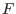depending on a set of parameters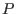and you want to find if there are values of these parameters such that the root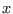of the polynomial verifies some properties. A natural way of doing that is to use the available generic analytical form of the root and check if you may find parameters such thatsatisfy the properties. In terms of interval analysis you have already transformed your problem and this may not be a good idea (the analytical form of the roots of a 3rd order polynomial is badly conditioned).

Rule: it is usually a bad idea to try to adapt to interval analysis the last step of a solving problem that has already been transformed to fit another method

Thinking backward what you want really is to verify if thesatisfying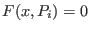satisfy the properties. Hence you should use as unknown not only thebut also the: you add an unknown but the calculation will then involve only the evaluation of the polynomial which is much more simple than the evaluation of the analytical form of the roots.

Rule: introducing new variables for simplifying expressions may be a good strategy

The rule for the right formulation is to find the right compromise between the complexity of the expressions (very complex expressions will usually lead to a large overestimation of the expressions) and the number of variables.

A good strategy is also to learn what are the main problems that have been addressed in interval analysis so that you can later on transform your problem into another one for which solving algorithms are available.

Rule: investigate which main problems have been addressed in interval analysis so that you may later on transform your problem into another one for which solving algorithms are available

Typical of such approach is to determine if a parametric matrix may include singular matrices. You may be tempted to derive the determinant of the interval matrix and then to determine if there are values of the unknowns that cancel it. But if you have not been able to manually determine these values this probably means that the determinant is quite complicated, and therefore not appropriate for interval analysis. But determining if a parametric matrix may include a singular matrix is a well-known problem in interval analysis and for solving this problem there are efficient methods that do not require the calculation of the determinant (see section 7.4).

Another case of failure occurs when the system is badly conditioned. This may occur, for example, when you have large coefficients in an equation while the values of the variable are very small: as ALIAS takes into account the round-off errors you will get large interval evaluation even for a small width of the intervals in the input interval (see for example the evaluation of the Wilkinson polynomial in section 5.9.3.2). There is no standard way to solve the problem. The first thing you may try is to normalize the unknowns in order to reduce the size of the coefficients. A second approach may be to switch a solving problem to an optimization problem.

Another trick for system solving is to combine the initial equations to get new one. For example consider the system:

```
cons1 := a1 + a2 + a3 + 1 ;
cons2 := a1 + 2*a2*x1 + 3*a3*x1^2 + 4*x1^3 ;
cons3 := a1 + 2*a2*x2 + 3*a3*x2^2 + 4*x2^3 ;
cons4 := a1 + 2*a2*x3 + 3*a3*x3^2 + 4*x3^3 ;
cons5 := a1*(x1+x2) + a2*(x1^2 + x2^2) + a3*(x1^3 + x2^3) + x1^4 + x2^4 ;
cons6 := a1*(x2+x3) + a2*(x2^2 + x3^2) + a3*(x2^3 + x3^3) + x2^4 + x3^4 ;
cons7 := x1 + delta -x2<= 0;
cons8 := x2 + delta -x3<= 0;
```
(which is the fredtest example of our bench). As it this system is difficult to solve (the computation time is over 10 minutes with a 3B increment of 0.001). Now we may notice that monomials involving a1 appear in many equations. Hence we consider adding as equations cons2-cons1, cons2-cons3 and so on. With this additional equations the computation time will be about 1mn 20 seconds.

Rule:Very often introducing additional constraints derived from the set of initial ones will reduce the solving time

Interval arithmetics plays evidently a large role in the computation time. The interval evaluation of mathematical operator such as sine, cosine,...is relatively expensive. If similar complex expressions appear more than once in an expression it is usually very efficient to compute it only once and to substitute it in the other places.

Rule:try to avoid multiple interval evaluation of the same complex expressions

### Choosing the right heuristics

In interval analysis we use a lot of heuristics (such as the use or not of the derivatives, the use of the 2B, 3B filtering methods,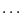) and finding the combination that will be the most efficient to solve a problem is a complex issue. Furthermore the choice of parameters of these heuristics (for example the step size in the 3B method) may have a very large influence on the computation time (and we mean a really large influence with a decrease factor of the computation time that may be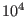).

In our experience for solving equation systems you have better always use the 2B and 3B methods (see sections 2.3.2 and 2.17). But this manual provides also numerous filters that may be more specific but also very efficient.

## Crash and bugs

You may have found an ALIAS bug either because the program crashes or because you get a wrong result (or no result at all while there is clearly at least one solution) or because the computation time is very large.

In the first case first check the error message you get (if any). If you get a message that originates from Bias/Profil such as Abort: Division by Zero this means that you have an expression whose interval evaluation is not allowed in some cases: see section 16.2.1 to determine how to avoid this problem. In the second case check with section 16.2.2 that you have correctly written your expressions. In the third case see if some method proposed in section 16.2.4 may help.

In all other cases we will be happy to have a look at your program and see what has gone wrong or what can be improved.

The bug/problem report should contain as much information as possible so that we can repeat your problem on our computers. Clearly this report should indicate what type of computer you are using, the operating system and the C++/Maple files that have been used. It may also be interesting to give some background information on the problem you want to solve. By giving background information on the problem we may also be able to suggest another, maybe simpler, way to solve it.

# Setting the debug option

If you cannot succeed in finding the right parameters you may use the debug option and to try to understand what is going on.

The algorithms of ALIAS are in general giving some information when running. There is a debug tool with three different levels: the first one (which is the default) is user-oriented and will help you to figure out what is going on, the second one is for expert and the third one is no debug at all. To set the debug option add to your program one of the following sentence:

```
Debug_Level_Solve_General_Interval=0;
Debug_Level_Solve_General_Interval=2;
```
or, if you are running ALIAS from MAPLE, add to your MAPLE program one of the following line:
```
`ALIAS/debug`:=2:
`ALIAS/debug`:=0:
```
The value 0 indicates no debug while level 2 is the highest (1 being the default). Then, when you run your program ALIAS will print lines looking like the following one:
```
Current box (11/23,remain:13), Sol: 0 (W=0.1,73.275)
```
This indicate that the algorithm is dealing with the box 11 in a list that has 23 elements. Thus 13 boxes are still to be considered as the algorithm will end when all the elements in the list have been considered.

The following part Sol:0 indicates that up to now ALIAS has found 0 solutions (for an optimization problem the value you get here is the current minima or maxima).

The last element, (W=0.1,73.275) indicates that for the current box the minimal width of the intervals in the set is 0.1 while the largest width is 73.275. With these informations you may figure out what is going on in the algorithm.Next: Contents Up: ALIAS-C++ Previous: Examples of application of